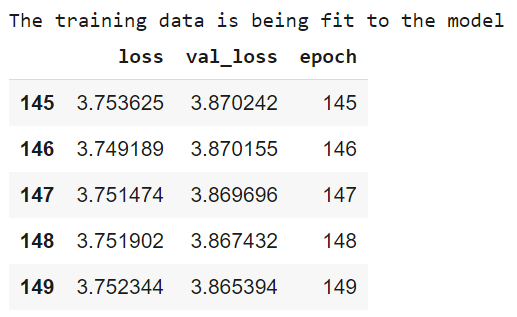# How can model be fit to data with Auto MPG dataset using TensorFlow?

Tensorflow is a machine learning framework that is provided by Google. It is an open−source framework used in conjunction with Python to implement algorithms, deep learning applications and much more. It is used in research and for production purposes.

The ‘tensorflow’ package can be installed on Windows using the below line of code −

pip install tensorflow

Tensor is a data structure used in TensorFlow. It helps connect edges in a flow diagram. This flow diagram is known as the ‘Data flow graph’. Tensors are nothing but multidimensional array or a list.

The aim behind a regression problem is to predict the output of a continuous or discrete variable, such as a price, probability, whether it would rain or not and so on.

The dataset we use is called the ‘Auto MPG’ dataset. It contains fuel efficiency of 1970s and 1980s automobiles. It includes attributes like weight, horsepower, displacement, and so on. With this, we need to predict the fuel efficiency of specific vehicles.

We are using the Google Colaboratory to run the below code. Google Colab or Colaboratory helps run Python code over the browser and requires zero configuration and free access to GPUs (Graphical Processing Units). Colaboratory has been built on top of Jupyter Notebook. Following is the code snippet −

## Example

print("The training data is being fit to the model ")
history = hrspwr_model.fit(
train_features['Horsepower'], train_labels,
epochs=150,
verbose=0,
validation_split = 0.3)

hist = pd.DataFrame(history.history)
hist['epoch'] = history.epoch
hist.tail()

## Output## Explanation

• The data is fit to the model using the ‘fit’ function.

• The number of steps is set using the ‘epochs’ attribute.

• The ‘history’ object stores the progress of the statistics associated with the input data.

• It is converted into a dataframe.

• A sample of the data is displayed on the console.

• The data is also visualized.